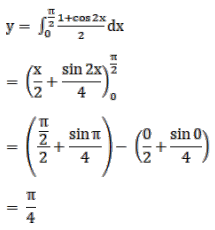# Mark against the correct answer in the following:

Question:

Mark $(\sqrt{)})$ against the correct answer in the following:

$\int_{0}^{\pi / 2} \cos ^{2} x d x=?$

A. $\frac{\pi}{2}$

B. $\pi$

C. $\frac{\pi}{4}$

D. 1

Solution: